小波与小波包、小波包分解与信号重构、小波包能量特征提取 暨 小波包分解后实现按频率大小分布重新排列(Matlab 程序详解）

-----暨 小波包分解后解决频率大小分布重新排列问题

本人当前对小波理解不是很深入，通过翻阅网络他人博客，进行汇总总结，重新调试Matlab代码，实现对小波与小波包、小波包分解与信号重构、小波包能量特征提取，供大家参考，后续将继续更新！

本人在分析信号的过程中发现，按照网上所述的小波包分解方法理解，获取每层节点重构后信号频率并不是按照（n,0）、(n,1)...顺序依次由小到大排列的，经过进一步分析研究后发现，需要对节点进行重排序，具体操作见本文分析。

1.小波与小波包区别

工程应用中经常需要对一些非平稳信号进行，小波分析和小波包分析适合对非平稳信号分析，相比较小波分析，利用小波包分析可以对信号分析更加精细，小波包分析可以将时频平面划分的更为细致，对信号的高频部分的分辨率要好于小波分析，可以根据信号的特征，自适应的选择最佳小波基函数，比便更好的对信号进行分析，所以小波包分析应用更加广泛。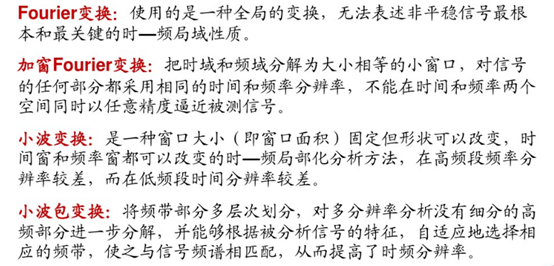①小波分解

小波变换只对信号的低频部分做进一步分解，而对高频部分也即信号的细节部分不再继续分解，所以小波变换能够很好地表征一大类以低频信息为主要成分的信号，不能很好地分解和表示包含大量细节信息（细小边缘或纹理）的信号，如非平稳机械振动信号、遥感图象、地震信号和生物医学信号等。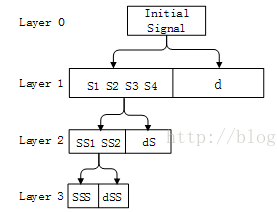②小波包分解

小波包变换既可以对低频部分信号进行分解，也可以对高频部分进行分解，而且这种分解既无冗余，也无疏漏，所以对包含大量中、高频信息的信号能够进行更好的时频局部化分析。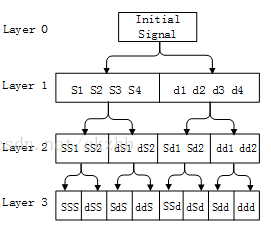2.小波包——小波包树与时频图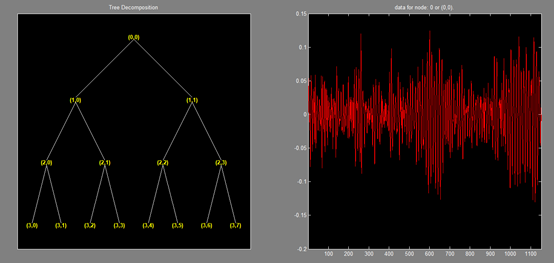以上即是小波包树，其中节点的命名规则是从（1，0）开始，叫1号， （1，1）是2号………依此类推，（3，0）是7号，（3，7）是14号。 每个节点都有对应的小波包系数，这个系数决定了频率的大小，也就是说频率信息已经有了，但是时域信息在哪里呢？ 那就是 order。  这个order就是这些节点的顺序，也就是频率的顺序。

Matlab实例：

clear all
clc
fs=1024;  %采样频率
f1=100;   %信号的第一个频率
f2=300;   %信号第二个频率
t=0:1/fs:1;
s=sin(2*pi*f1*t)+sin(2*pi*f2*t);  %生成混合信号
[tt]=wpdec(s,3,'dmey');  %小波包分解，3代表分解3层，'dmey'使用meyr小波
plot(tt)               %画小波包树图
wpviewcf(tt,1);        %画出时间频率图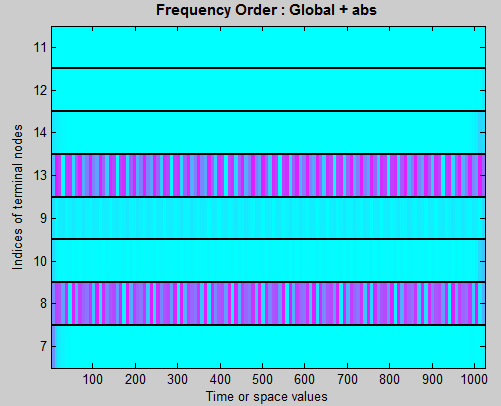x轴，就是1024个点，对应1秒，每个点就代表1/1024秒。

y轴，显示的数字对应于小波包树中的节点，从下面开始，顺序是 7号节点，8号，10号，9号，，，，11号节点，这个顺序是这么排列的，这是小波包自动排列的。然后，y轴是频率啊，怎么不是 100Hz和300Hz呢？我们的采样频率是1024Hz，根据采样定理，奈奎斯特采样频率是512Hz，我们分解了3层，最后一层就是 2^3=8个频率段，每个频率段的频率区间是 512/8=64Hz,看图颜色重的地方一个是在8那里，一个在13那里，8是第二段，也就是 65-128Hz之间，13是第五段，也就是257-320Hz之间。这样就说通了，正好这个原始信号只有两个频率段，一个100一个300。如果我们不是分解了3层，而是更多层，那么每个频率段包含的频率也就越窄，图上有颜色的地方也会更细，也就是说更精细了，由于原始信号的频率在整个1秒钟内都没有改变，所以有颜色的地方是一个横线。（引用：http://www.cnblogs.com/welen/articles/5667217.html ）

3.小波包-----小波包分解系数

在数值分析中，我们学过内积，内积的物理含义：两个图形的相似性，若两个图形完全正交，则内积为0，若两个图形完全一样，则系数为1（相对值）。小波变换的实质是：原信号与小波基函数的相似性。小波系数就是小波基函数原信号相似的系数

连续小波变换：小波函数与原信号对应点相乘，再相加，得到对应点的小波变换系数，平移小波基函数，再计算小波函数与原信号对应点相乘，再相加，这样就得到一系列的小波系数。对于离散小波变换（由于很多小波函数不是正交函数，因此需要一个尺度函数）所以，原信号函数可以分解成尺度函数和小波函数的线性组合，在这个函数中，尺度函数产生低频部分，小波函数产生高频部分。

4.小波包-----信号分解与重构（方法1）

1

t=wpdec(wave,3,'dmey');
t2 = wpjoin(t,[3;4;5;6]);
cfs3  = zeros(sNod(1,:));
cfs4  = zeros(sNod(2,:));
cfs5  = zeros(sNod(3,:));
cfs6  = zeros(sNod(4,:));
t3 = write(t2,'cfs',3,cfs3,'cfs',4,cfs4,'cfs',5,cfs5,'cfs',6,cfs6);
wave2=wprec(t3);


可以预见，因为我们把小波树的节点系数都变为0了，所以信号也就全为0了。所以wave2是一个0向量。读者可以自行plot一下wave和wave2看看。进一步，如果我们只聚合第二层中的某几个节点，比如 4和5，即将第三行到第八行中节点 3 和节点 6的语句删除或修改，那么意思就是将 4  5节点的系数变为0，那么wave2肯定就不是0向量了。

2

t=wpdec(wave,3,'dmey');
t2 = wpjoin(t,[3;4;5;6]);
cfs3=wpcoef(t,3);
cfs4=wpcoef(t,4);
cfs5=wpcoef(t,5);
cfs6=wpcoef(t,6);
t3 = write(t2,'cfs',3,cfs3,'cfs',4,cfs4,'cfs',5,cfs5,'cfs',6,cfs6);
wave2=wprec(t3);


3

t=wpdec(wave,3,'dmey');
cfs7=wpcoef(t,7);
cfs8=wpcoef(t,8);
cfs9=wpcoef(t,9);
cfs10=wpcoef(t,10);
cfs11=wpcoef(t,11);
cfs12=wpcoef(t,12);
cfs13=wpcoef(t,13);
cfs14=wpcoef(t,14);
t3=write(t,'cfs',7,cfs7,'cfs',8,cfs8,'cfs',9,cfs9,'cfs',10,cfs10,'cfs',11,cfs11,'cfs',...
12,cfs12,'cfs',13,cfs13,'cfs',14,cfs14);
y=wprec(t3);


5.小波包-----信号分解与重构（方法2）

x_input=x_train(:,1,1);                  %输入数据
plot(x_input);title('输入信号时域图像')   %绘制输入信号时域图像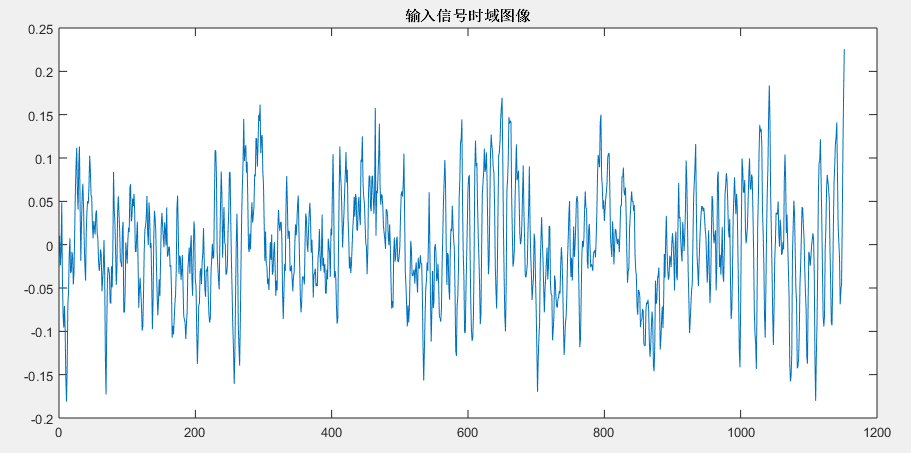%%   查看频谱范围
x=x_input;
fs=128;
N=length(x); %采样点个数
signalFFT=abs(fft(x,N));%真实的幅值
Y=2*signalFFT/N;
f=(0:N/2)*(fs/N);
figure;plot(f,Y(1:N/2+1));
ylabel('amp'); xlabel('frequency');title('输入信号的频谱');grid on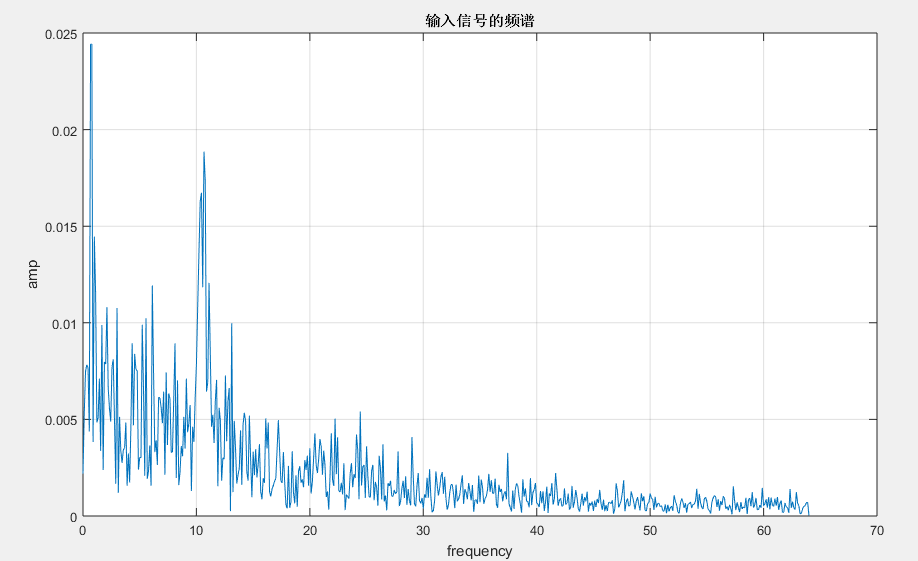wpt=wpdec(x_input,3,'dmey');        %进行3层小波包分解
plot(wpt);                          %绘制小波包树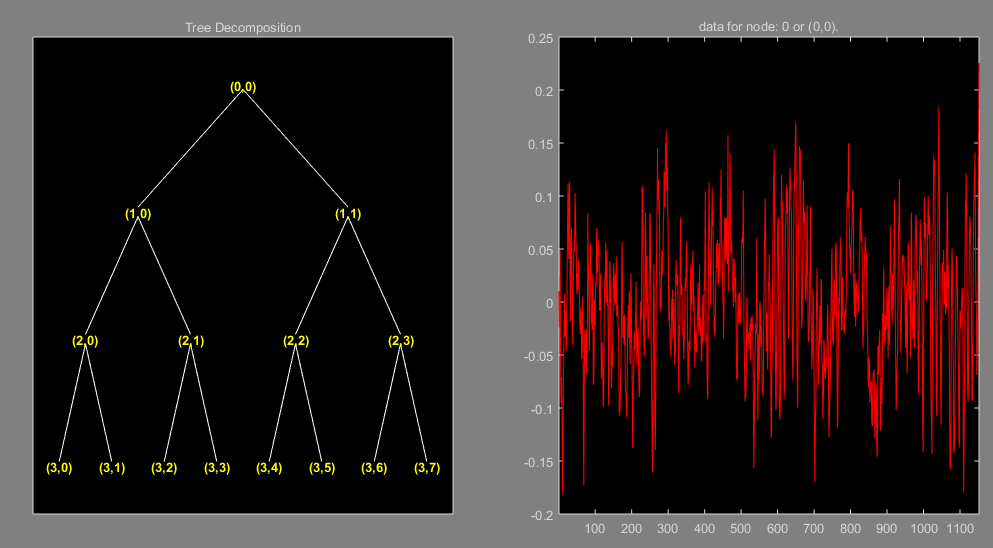for i=0:7
rex3(:,i+1)=wprcoef(wpt,[3 i]);  %实现对节点小波节点进行重构
end

figure;                          %绘制第3层各个节点分别重构后信号的频谱
for i=0:7
subplot(2,4,i+1);
x_sign=rex3(:,i+1);
N=length(x_sign); %采样点个数
signalFFT=abs(fft(x_sign,N));%真实的幅值
Y=2*signalFFT/N;
f=(0:N/2)*(fs/N);
plot(f,Y(1:N/2+1));
ylabel('amp'); xlabel('frequency');grid on
axis([0 50 0 0.03]); title(['小波包第3层',num2str(i),'节点信号频谱']);
end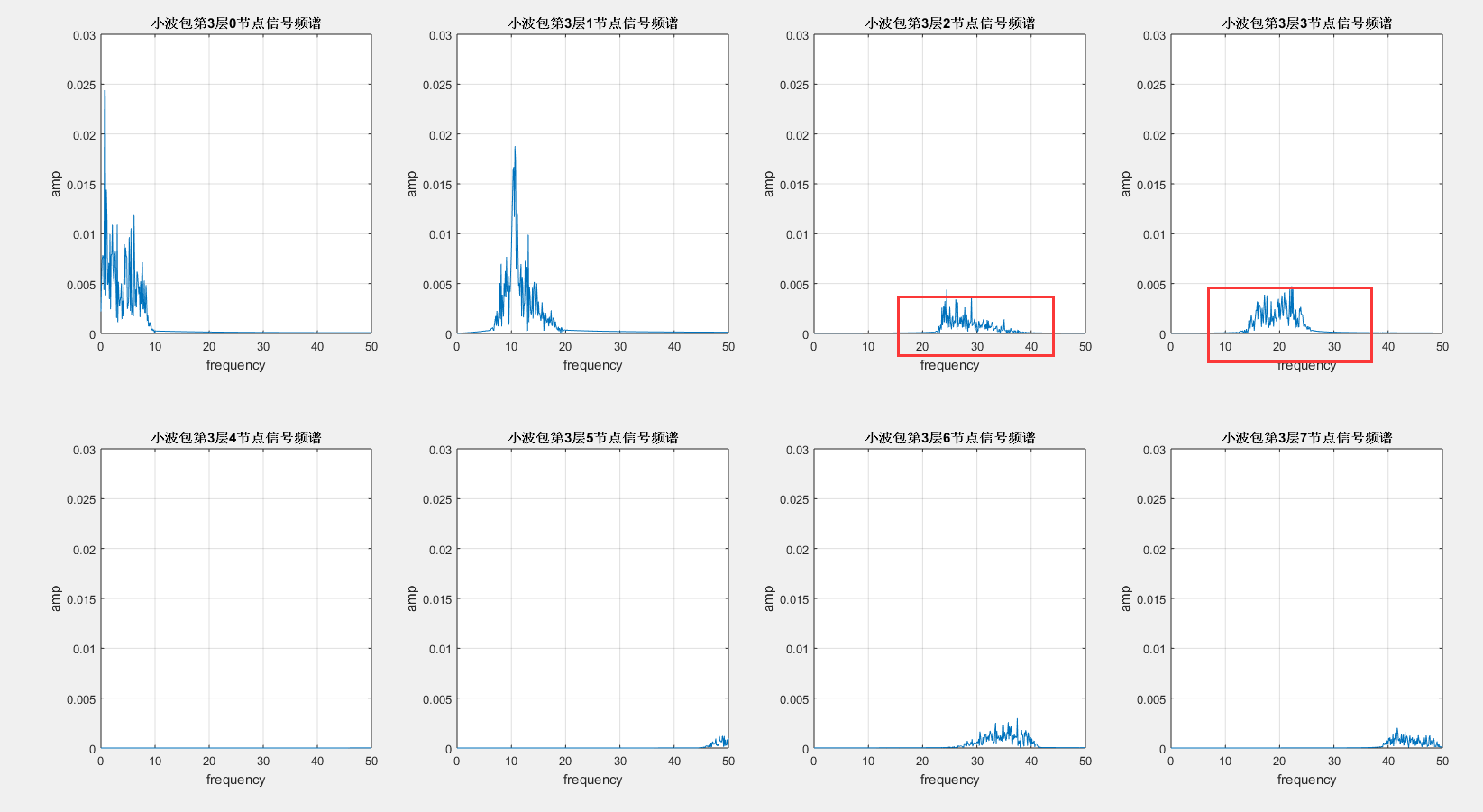nodes=[7;8;9;10;11;12;13;14];   %第3层的节点号
ord=wpfrqord(nodes);  %小波包系数重排，ord是重排后小波包系数索引构成的矩阵　如3层分解的[1;2;4;3;7;8;6;5]
nodes_ord=nodes(ord); %重排后的小波系数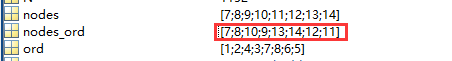for i=1:8
rex3(:,i)=wprcoef(wpt,nodes_ord(i));  %实现对节点小波节点进行重构
end

figure;                         %绘制第3层各个节点分别重构后信号的频谱
for i=0:7
subplot(2,4,i+1);
x_sign= rex3(:,i+1);
N=length(x_sign); %采样点个数
signalFFT=abs(fft(x_sign,N));%真实的幅值
Y=2*signalFFT/N;
f=(0:N/2)*(fs/N);
plot(f,Y(1:N/2+1));
ylabel('amp'); xlabel('frequency');grid on
axis([0 50 0 0.03]); title(['小波包第3层',num2str(i),'节点信号频谱']);
end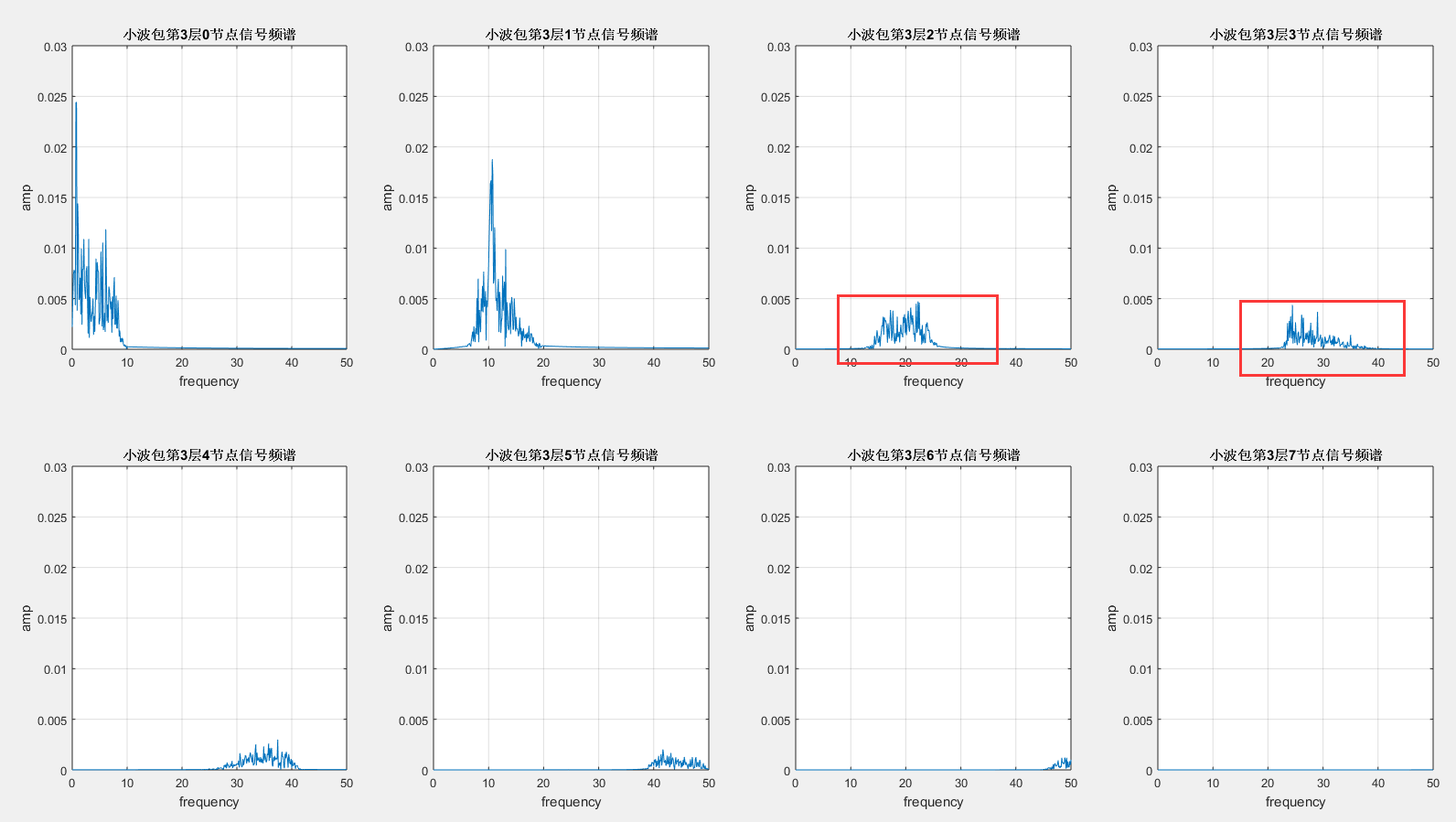6.小波包分解------能量特征提取（方法1）

%% wavelet packet coefficients. 求取小波包分解的各个节点的小波包系数
cfs3_0=wpcoef(wpt,nodes_ord(1));  %对重排序后第3层0节点的小波包系数0-8Hz
cfs3_1=wpcoef(wpt,nodes_ord(2));  %对重排序后第3层0节点的小波包系数8-16Hz
cfs3_2=wpcoef(wpt,nodes_ord(3));  %对重排序后第3层0节点的小波包系数16-24Hz
cfs3_3=wpcoef(wpt,nodes_ord(4));  %对重排序后第3层0节点的小波包系数24-32Hz
cfs3_4=wpcoef(wpt,nodes_ord(5));  %对重排序后第3层0节点的小波包系数32-40Hz
cfs3_5=wpcoef(wpt,nodes_ord(6));  %对重排序后第3层0节点的小波包系数40-48Hz
cfs3_6=wpcoef(wpt,nodes_ord(7));  %对重排序后第3层0节点的小波包系数48-56Hz
cfs3_7=wpcoef(wpt,nodes_ord(8));  %对重排序后第3层0节点的小波包系数56-64Hz

E_cfs3_0=norm(cfs3_0,2)^2;  %% 1-范数：就是norm(...,1)，即各元素绝对值之和；2-范数：就是norm(...,2)，即各元素平方和开根号；
E_cfs3_1=norm(cfs3_1,2)^2;
E_cfs3_2=norm(cfs3_2,2)^2;
E_cfs3_3=norm(cfs3_3,2)^2;
E_cfs3_4=norm(cfs3_4,2)^2;
E_cfs3_5=norm(cfs3_5,2)^2;
E_cfs3_6=norm(cfs3_6,2)^2;
E_cfs3_7=norm(cfs3_7,2)^2;
E_total=E_cfs3_0+E_cfs3_1+E_cfs3_2+E_cfs3_3+E_cfs3_4+E_cfs3_5+E_cfs3_6+E_cfs3_7;

p_node(0)= 100*E_cfs3_0/E_total;           % 求得每个节点的占比
p_node(2)= 100*E_cfs3_1/E_total;           % 求得每个节点的占比
p_node(3)= 100*E_cfs3_2/E_total;           % 求得每个节点的占比
p_node(4)= 100*E_cfs3_3/E_total;           % 求得每个节点的占比
p_node(5)= 100*E_cfs3_4/E_total;           % 求得每个节点的占比
p_node(6)= 100*E_cfs3_5/E_total;           % 求得每个节点的占比
p_node(7)= 100*E_cfs3_6/E_total;           % 求得每个节点的占比
p_node(8)= 100*E_cfs3_7/E_total;           % 求得每个节点的占比

figure;
x=1:8;
bar(x,p_node);
title('各个频段能量所占的比例');
xlabel('频率 Hz');
ylabel('能量百分比/%');
for j=1:8
text(x(j),p_node(j),num2str(p_node(j),'%0.2f'),...
'HorizontalAlignment','center',...
'VerticalAlignment','bottom')
end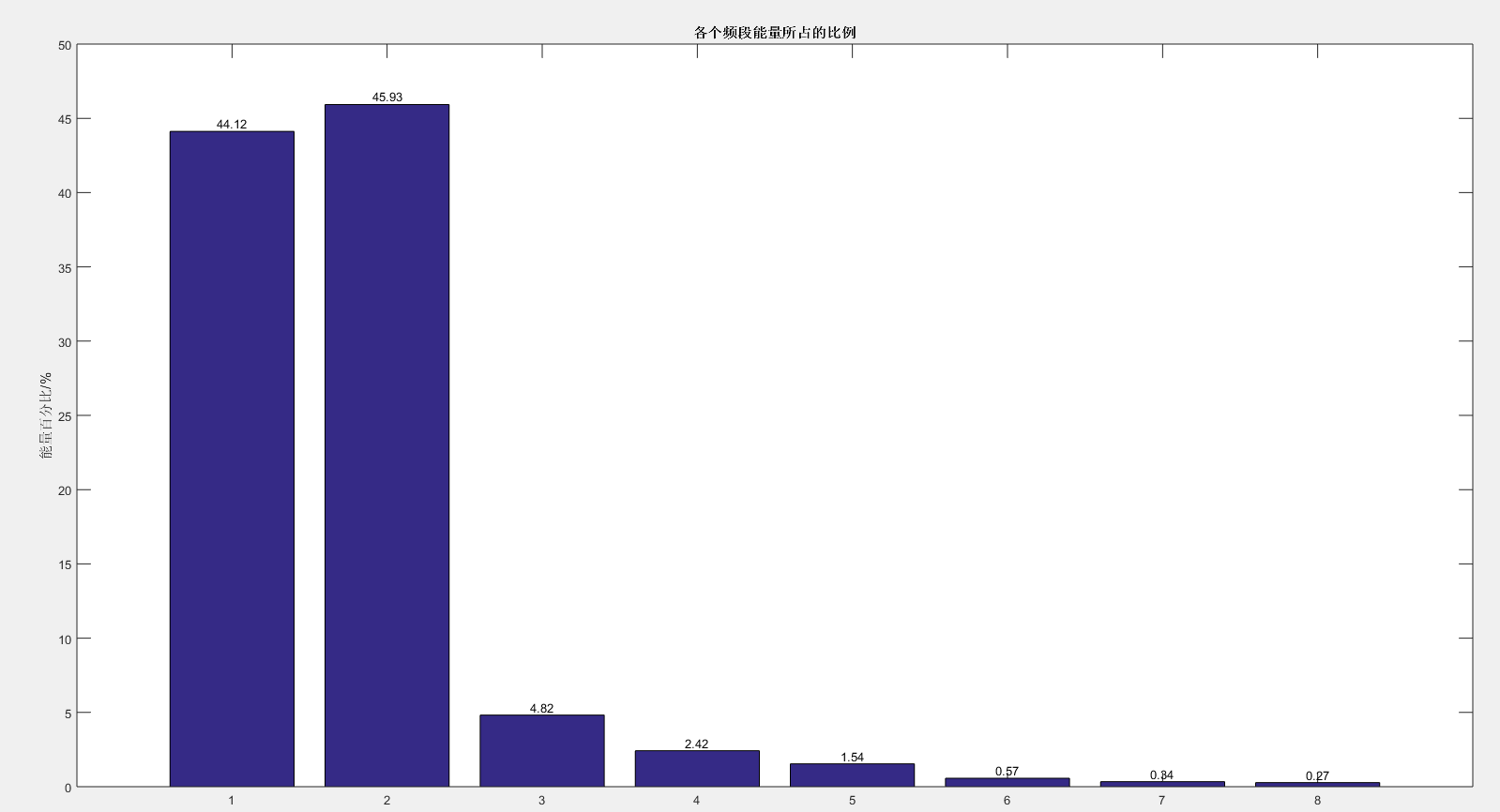7.小波包分解------能量特征提取（方法2）

E = wenergy(wpt);   %该函数只能对最后一层（底层）节点进行能量提取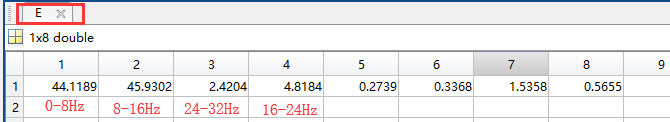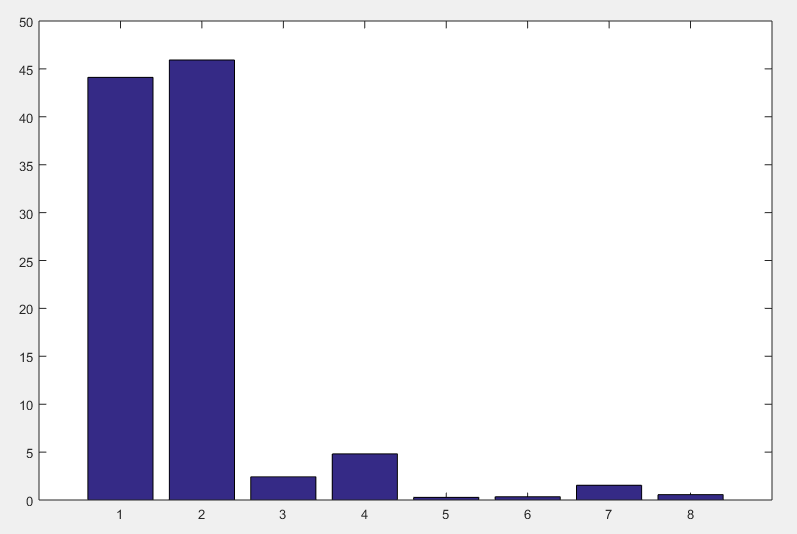%% 绘制重构前各个频段小波包系数
figure(1);
subplot(4,1,1);
plot(x_input);
title('原始信号');
subplot(4,1,2);
plot(cfs3_0);
title(['层数 ',num2str(3) '  节点 0的小波0-8Hz',' 系数'])
subplot(4,1,3);
plot(cfs3_1);
title(['层数 ',num2str(3) '  节点 1的小波8-16Hz',' 系数'])
subplot(4,1,4);
plot(cfs3_2);
title(['层数 ',num2str(3) '  节点 2的小波16-24Hz',' 系数'])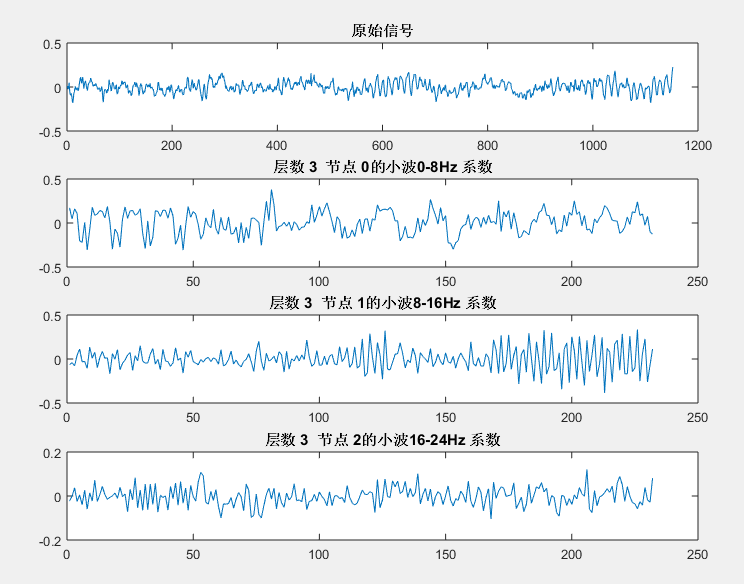%% 绘制重构后时域各个特征频段的图形
figure(3);
subplot(3,1,1);
plot(rex3(:,1));
title('重构后0-8Hz频段信号');
subplot(3,1,2);
plot(rex3(:,2));
title('重构后8-16Hz频段信号')
subplot(3,1,3);
plot(rex3(:,3));
title('重构后16-24Hz频段信号');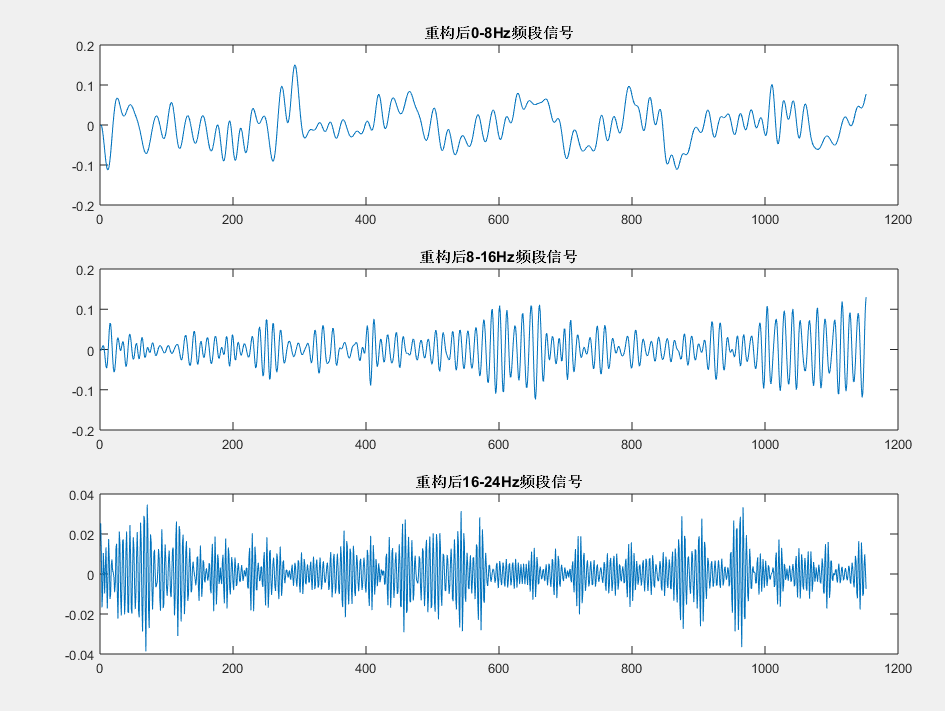clear all;

x_input=x_train(:,1,1);           %输入数据
plot(x_input);title('输入信号时域图像')  %绘制输入信号时域图像

x=x_input;        %   查看频谱范围
fs=128;
N=length(x); %采样点个数
signalFFT=abs(fft(x,N));%真实的幅值
Y=2*signalFFT/N;
f=(0:N/2)*(fs/N);
figure;plot(f,Y(1:N/2+1));
ylabel('amp'); xlabel('frequency');title('输入信号的频谱');grid on

wpt=wpdec(x_input,3,'dmey');      %进行4层小波包分解
plot(wpt);                        %绘制小波包树

%% 实现对节点顺序按照频率递增进行重排序
% nodes=get(wpt,'tn');  %小波包分解系数　为什么ｎｏｄｅｓ是[7;8;9;10;11;12;13;14]
% N_cfs=length(nodes);  %小波包系数个数
nodes=[7;8;9;10;11;12;13;14];
ord=wpfrqord(nodes);  %小波包系数重排，ord是重排后小波包系数索引构成的矩阵　如3层分解的[1;2;4;3;7;8;6;5]
nodes_ord=nodes(ord); %重排后的小波系数

%% 实现对节点小波节点进行重构
for i=1:8
rex3(:,i)=wprcoef(wpt,nodes_ord(i));
end

%% 绘制第3层各个节点分别重构后信号的频谱
figure;
for i=0:7
subplot(2,4,i+1);
x_sign= rex3(:,i+1);
N=length(x_sign); %采样点个数
signalFFT=abs(fft(x_sign,N));%真实的幅值
Y=2*signalFFT/N;
f=(0:N/2)*(fs/N);
plot(f,Y(1:N/2+1));
ylabel('amp'); xlabel('frequency');grid on
axis([0 50 0 0.03]); title(['小波包第3层',num2str(i),'节点信号频谱']);
end

%% wavelet packet coefficients. 求取小波包分解的各个频段的小波包系数
cfs3_0=wpcoef(wpt,nodes_ord(1));  %对重排序后第3层0节点的小波包系数0-8Hz
cfs3_1=wpcoef(wpt,nodes_ord(2));  %对重排序后第3层0节点的小波包系数8-16Hz
cfs3_2=wpcoef(wpt,nodes_ord(3));  %对重排序后第3层0节点的小波包系数16-24Hz
cfs3_3=wpcoef(wpt,nodes_ord(4));  %对重排序后第3层0节点的小波包系数24-32Hz
cfs3_4=wpcoef(wpt,nodes_ord(5));  %对重排序后第3层0节点的小波包系数32-40Hz
cfs3_5=wpcoef(wpt,nodes_ord(6));  %对重排序后第3层0节点的小波包系数40-48Hz
cfs3_6=wpcoef(wpt,nodes_ord(7));  %对重排序后第3层0节点的小波包系数48-56Hz
cfs3_7=wpcoef(wpt,nodes_ord(8));  %对重排序后第3层0节点的小波包系数56-64Hz
%% 求取小波包分解的各个频段的能量
E_cfs3_0=norm(cfs3_0,2)^2;  %% 1-范数：就是norm(...,1)，即各元素绝对值之和；2-范数：就是norm(...,2)，即各元素平方和开根号；
E_cfs3_1=norm(cfs3_1,2)^2;
E_cfs3_2=norm(cfs3_2,2)^2;
E_cfs3_3=norm(cfs3_3,2)^2;
E_cfs3_4=norm(cfs3_4,2)^2;
E_cfs3_5=norm(cfs3_5,2)^2;
E_cfs3_6=norm(cfs3_6,2)^2;
E_cfs3_7=norm(cfs3_7,2)^2;
E_total=E_cfs3_0+E_cfs3_1+E_cfs3_2+E_cfs3_3+E_cfs3_4+E_cfs3_5+E_cfs3_6+E_cfs3_7;

p_node(0)= 100*E_cfs3_0/E_total;           % 求得每个节点的占比
p_node(2)= 100*E_cfs3_1/E_total;           % 求得每个节点的占比
p_node(3)= 100*E_cfs3_2/E_total;           % 求得每个节点的占比
p_node(4)= 100*E_cfs3_3/E_total;           % 求得每个节点的占比
p_node(5)= 100*E_cfs3_4/E_total;           % 求得每个节点的占比
p_node(6)= 100*E_cfs3_5/E_total;           % 求得每个节点的占比
p_node(7)= 100*E_cfs3_6/E_total;           % 求得每个节点的占比
p_node(8)= 100*E_cfs3_7/E_total;           % 求得每个节点的占比

figure;
x=1:8;
bar(x,p_node);
title('各个频段能量所占的比例');
xlabel('频率 Hz');
ylabel('能量百分比/%');
for j=1:8
text(x(j),p_node(j),num2str(p_node(j),'%0.2f'),...
'HorizontalAlignment','center',...
'VerticalAlignment','bottom')
end
% E = wenergy(wpt);       %求取各个节点能量

%% 绘制重构前各个特征频段小波包系数的图形
figure(1);
subplot(4,1,1);
plot(x_input);
title('原始信号');
subplot(4,1,2);
plot(cfs3_0);
title(['层数 ',num2str(3) '  节点 0的小波0-8Hz',' 系数'])
subplot(4,1,3);
plot(cfs3_1);
title(['层数 ',num2str(3) '  节点 1的小波8-16Hz',' 系数'])
subplot(4,1,4);
plot(cfs3_2);
title(['层数 ',num2str(3) '  节点 2的小波16-24Hz',' 系数'])

%% 绘制重构后时域各个特征频段的图形
figure(3);
subplot(3,1,1);
plot(rex3(:,1));
title('重构后0-8Hz频段信号');
subplot(3,1,2);
plot(rex3(:,2));
title('重构后8-16Hz频段信号')
subplot(3,1,3);
plot(rex3(:,3));
title('重构后16-24Hz频段信号');



8.小波----常见基函数

与标准的傅里叶变换相比，小波分析中使用到的小波函数具有不唯一性，即小波函数 具有多样性。小波分析在工程应用中，一个十分重要的问题就是最优小波基的选择问题，因为用不同的小波基分析同一个问题会产生不同的结果。目前我们主要是通过用小波分析方法处理信号的结果与理论结果的误差来判定小波基的好坏，由此决定小波基。

常用小波基有Haar小波、Daubechies(dbN)小波、Mexican Hat(mexh)小波、Morlet小波、Meyer小波等。

1.Haar小波Haar函数是小波分析中最早用到的一个具有紧支撑的正交小波函数，也是最简单的一个小波函数，它是支撑域在范围内的单个矩形波。Haar函数的定义如下：Haar小波在时域上是不连续的，所以作为基本小波性能不是特别好。但它也有自己的优点:计算简单。不但与正交，而且与自己的整数位移正交，因此，在的多分辨率系统中，Haar小波构成一组最简单的正交归一的小波族。

  [phi,g1,xval] = wavefun('haar',20);
subplot(2,1,1);
plot(xval,g1,'LineWidth',2);
xlabel('t')；
title('haar 时域');
g2=fft(g1);
g3=abs(g2);
subplot(2,1,2);
plot(g3,'LineWidth',2);
xlabel('f')title('haar 频域')；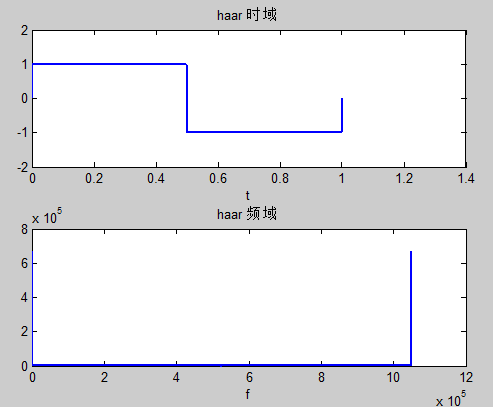2.Daubechies(dbN)小波Daubechies小波是世界著名的小波分析学者Inrid·Daubechies构造的小波函数，简写为dbN，N是小波的阶数。小波和尺度函数中的支撑区为的消失矩为。除（Harr小波）外，dbN不具有对称性（即非线性相位）。除（Harr小波）外，dbN没有明确的表达式，但转换函数h的平方模是明确的:令，其中为二项式的系数，则有其中：Daubechies小波具有以下特点：在时域是有限支撑的，即长度有限。在频域在处有N阶零点。和它的整数位移正交归一，即。小波函数可以由所谓“尺度函数”求出来。尺度函数为低通函数，长度有限，支撑域在的范围内。

 db4的时域和频域波形：
[phi,g1,xval] = wavefun('db4',10);
subplot(2,1,1);
plot(xval,g1,'LineWidth',2);
xlabel('t')title('db4 时域');
g2=fft(g1);
g3=abs(g2);
subplot(2,1,2);
plot(g3,'LineWidth',2);
xlabel('f')title('db4 频域')；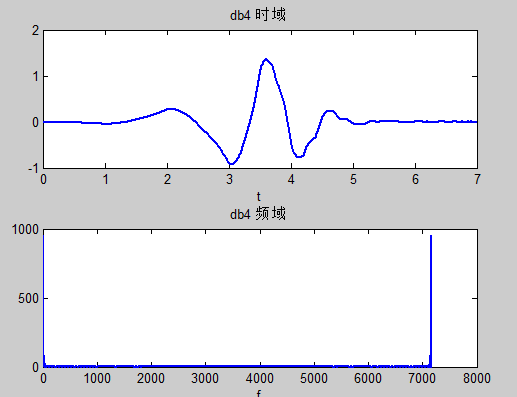Daubechies小波常用来分解和重构信号，作为滤波器使用:
[Lo_D,Hi_D,Lo_R,Hi_R] = wfilters('db4'); %计算该小波的4个滤波器
subplot(2,2,1);
stem(Lo_D,'LineWidth',2);
title('分解低通滤波器');
subplot(2,2,2);
stem(Hi_D,'LineWidth',2);
title('分解高通滤波器');
subplot(2,2,3);
stem(Lo_R,'LineWidth',2);
title('重构低通滤波器');
subplot(2,2,4);
stem(Hi_R,'LineWidth',2);
title('重构高通滤波器');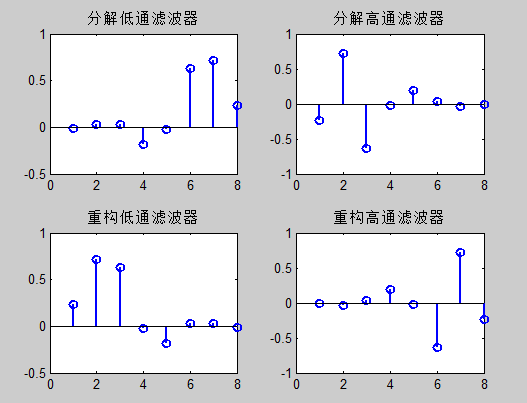3.Mexican Hat(mexh)小波Mexican Hat函数为Gauss函数的二阶导数：因为它的形状像墨西哥帽的截面，所以也称为墨西哥帽函数。Mexihat小波的时域和频域波形：

  Mexihat小波的时域和频域波形：
d=-6; h=6; n=100;
[g1,x]=mexihat(d,h,n);
subplot(2,1,1);
plot(x,g1,'LineWidth',2);
xlabel('t');
title('Mexihat 时域');
g2=fft(g1);
g3=(abs(g2));
subplot(2,1,2);
plot(g3,'LineWidth',2);
xlabel('f');
title('mexihat 频域');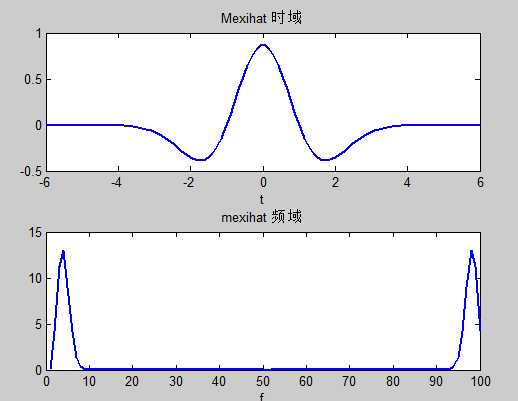Mexihat小波的特点：在时间域与频率域都有很好的局部化，并且满足。不存在尺度函数，所以Mexihat小波函数不具有正交性。

4.Morlet小波它是高斯包络下的单频率副正弦函数：其中C是重构时的归一化常数。Morlet小波没有尺度函数，而且是非正交分解。Morlet小波的时域和频域波形图：

  d=-6; h=6; n=100;
[g1,x]=morlet(d,h,n);
subplot(2,1,1);
plot(x,g1,'LineWidth',2);
xlabel('t');
title('morlet 时域');
g2=fft(g1);
g3=(abs(g2));
subplot(2,1,2);
plot(g3,'LineWidth',2);
xlabel('f');
title('mexihat 频域');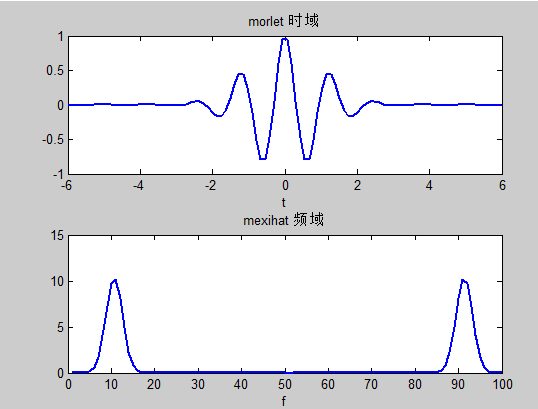注:

Wpcoef 是求解某个节点的小波包系数，数据长度是1/(2^n)（分解n层的话），其实就是将原始信号化成2^N段，每段的长度是相等的且比原信号短

wprcoef是把某个节点的小波包系数重构，得到的是和原信号一样长度的信号。

https://zhuanlan.zhihu.com/p/22450818

https://zhuanlan.zhihu.com/p/19763358

https://www.jianshu.com/p/5b5c160c7e3a